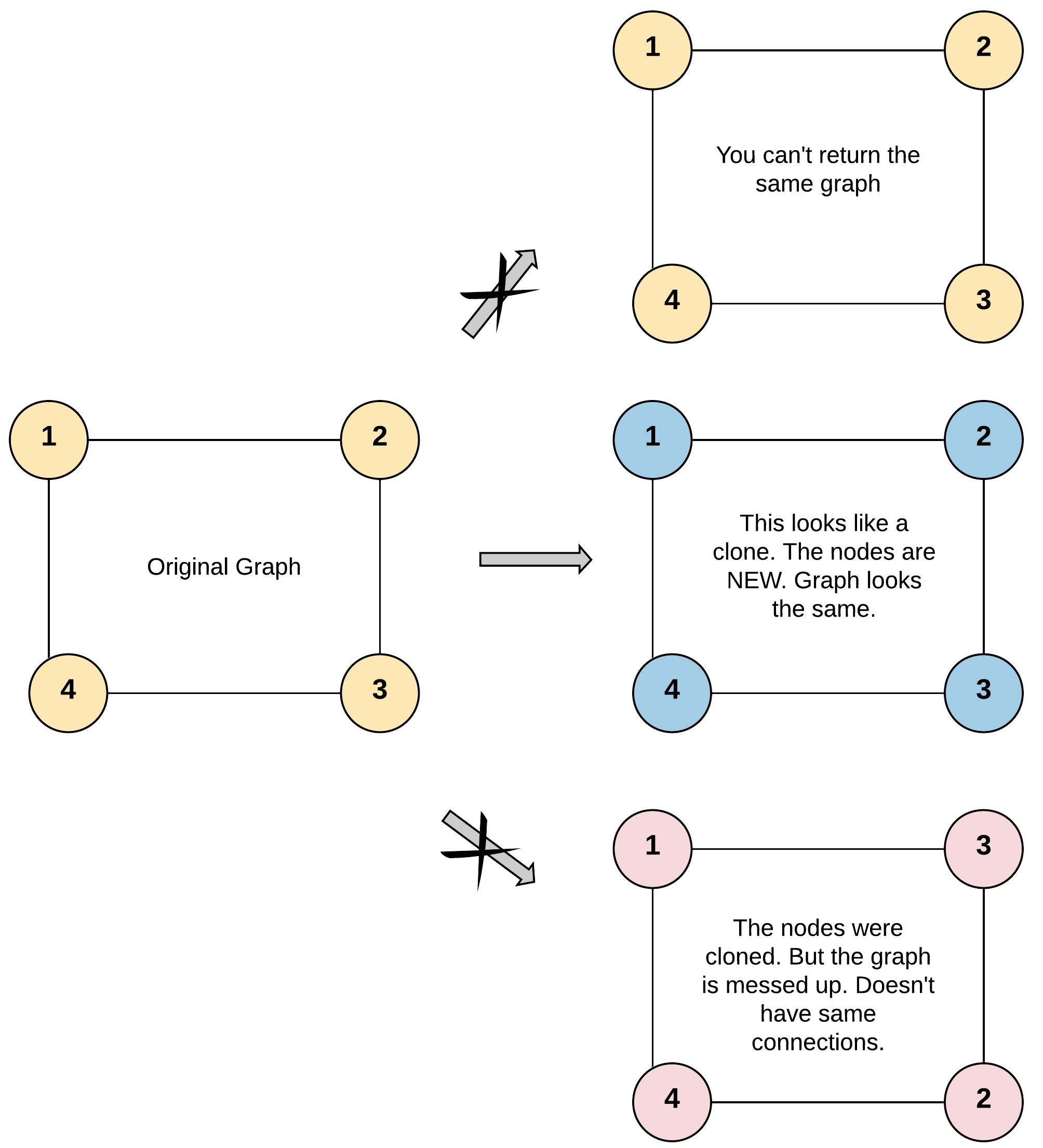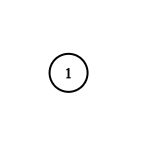# Clone Graph LeetCode Solution

Difficulty Level Medium
WixViews 41

## Problem Statement

Clone Graph LeetCode Solution – We are given a reference of a node in a connected undirected graph and are asked to return a deep copy of the graph. A deep copy is basically a clone where no node present in the deep copy should have the reference to any original graph node.

## Examples & Explanations

Example 1:```Input: adjList = [[2,4],[1,3],[2,4],[1,3]]
Output: [[2,4],[1,3],[2,4],[1,3]]
Explanation: There are 4 nodes in the graph.
1st node (val = 1)'s neighbors are 2nd node (val = 2) and 4th node (val = 4).
2nd node (val = 2)'s neighbors are 1st node (val = 1) and 3rd node (val = 3).
3rd node (val = 3)'s neighbors are 2nd node (val = 2) and 4th node (val = 4).
4th node (val = 4)'s neighbors are 1st node (val = 1) and 3rd node (val = 3).```

Example 2:```Input: adjList = [[]]
Output: [[]]
Explanation: Note that the input contains one empty list. The graph consists of only one node with val = 1 and it does not have any neighbors.```

Example 3:
```Input: adjList = []
Output: []
Explanation: This an empty graph, it does not have any nodes.```

## Approach

The idea is to create an empty undirected graph. We then run DFS on the original graph. If we encounter a node that is not present in the clone, we create a new node having the same value and add it to the clone. If it is already present then simply link the required edge. Here, we have used a hashmap “vis” of type linked list, where the key will be the node referenced in the original graph and the value will be the cloned node of the original node.

## Code

### C++ code for Clone Graph

```/*
// Definition for a Node.
class Node {
public:
int val;
vector<Node*> neighbors;
Node() {
val = 0;
neighbors = vector<Node*>();
}
Node(int _val) {
val = _val;
neighbors = vector<Node*>();
}
Node(int _val, vector<Node*> _neighbors) {
val = _val;
neighbors = _neighbors;
}
};
*/

class Solution {
map<Node*, Node*> vis;
Node* dfs(Node* node) {
// if the node is NULL simply return NULL
if(node == NULL) {
return node;
}
// if the node is already present in the vis, then return reference of that node
if(vis.find(node) != vis.end()) return vis[node];

// make a new node and store the value of original node
Node* newNode = new Node(node->val);
vis[node] = newNode;

// traverse through neighbors of node and push back dfs of each neighbor
for(Node* edges: node->neighbors) {
newNode->neighbors.push_back(dfs(edges));
}
return newNode;
}
public:
Node* cloneGraph(Node* node) {
Node* clone = dfs(node);
return clone;
}
};```

### Java code for Clone Graph

```/*
// Definition for a Node.
class Node {
public int val;
public List<Node> neighbors;
public Node() {
val = 0;
neighbors = new ArrayList<Node>();
}
public Node(int _val) {
val = _val;
neighbors = new ArrayList<Node>();
}
public Node(int _val, ArrayList<Node> _neighbors) {
val = _val;
neighbors = _neighbors;
}
}
*/

class Solution {
HashMap<Node,Node> vis = new HashMap<Node,Node>();
public Node dfs(Node node) {
// if the node is NULL simply return NULL
if(node == null) {
return node;
}
// if the node is already present in the vis, then return reference of that node
if(vis.containsKey(node)) return vis.get(node);

// make a new node and store the value of original node
Node newNode = new Node(node.val);
vis.put(node, newNode);

// traverse through neighbors of node and push back dfs of each neighbor
for(Node edges: node.neighbors) {
}
return newNode;
}
public Node cloneGraph(Node node) {
Node clone=dfs(node);
return clone;
}
}```

## Complexity Analysis for Clone Graph LeetCode Solution

• Time ComplexityO(N+M), where N is a number of nodes (vertices) and M is a number of edges.
• DFS takes O(N+M) time
• Space ComplexityO(N).
• The space required by hash map “vis” will also be occupied by the recursion stack in running DFS on the given graph.
• Let H be the height of the given graph, recursion stack will take O(H) space
• Total, this will occupy O(N) space
Translate »